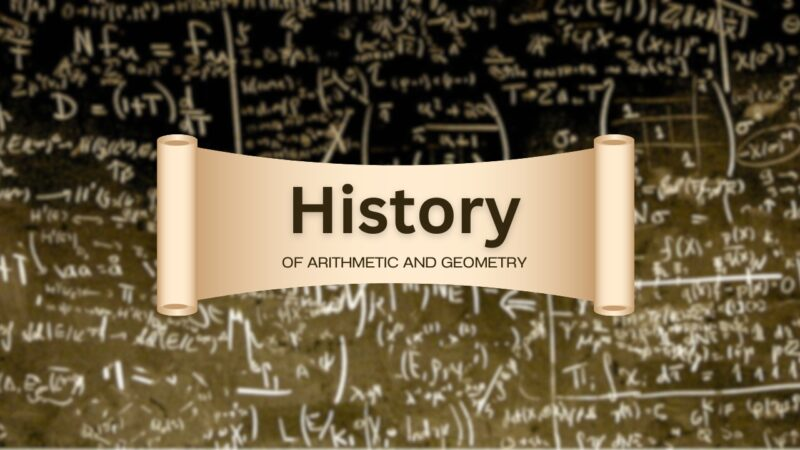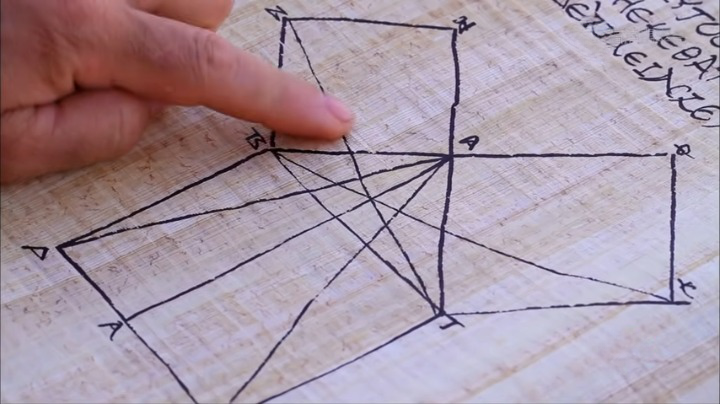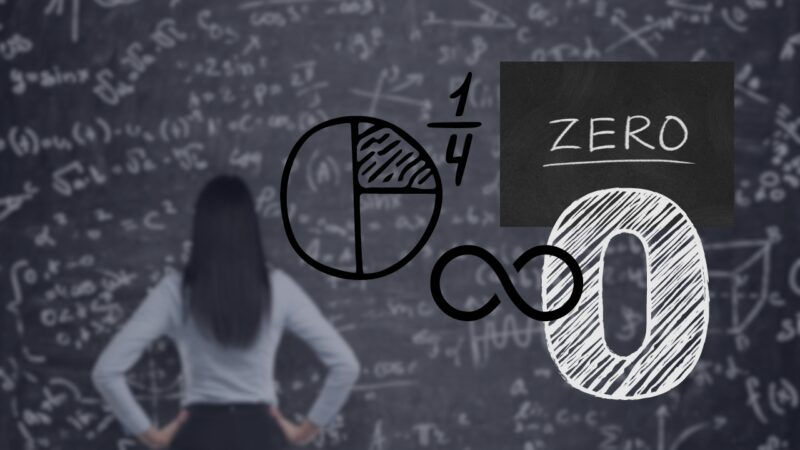# Who Invented Math: History, Facts and Table of Numerals

From the intricate geometric designs of ancient Egypt to the complex algorithms that drive today’s digital world, mathematics has shaped civilizations, underpinned discoveries, and made sense of the universe. But who can we credit for inventing mathematics?

Mathematics didn’t just appear out of nowhere; it evolved over centuries, varying among different cultures and epochs.  The origins of mathematics are a subject of ongoing research and debate.

Early human societies recognized the necessity of basic arithmetic and geometric principles for survival – for trading, land measurement, architecture, and astronomical observation. But pinning down the precise time and place of the inception of mathematics remains elusive.

## Pioneers of Arithmetic and Geometry### The Dawn of Arithmetic

Historically, the earliest evidence of mathematical knowledge dates back to the ancient Sumerians of Mesopotamia around 4,000 BCE. Sumerians used a sexagesimal (base-60) number system, which is the foundation for the way we measure time today.

Sumerians developed this number system to facilitate trade, land division, and taxation. They inscribed these mathematical concepts on clay tablets, giving us a glimpse into the origins of arithmetic.

### Geometry in Land Measurement

In addition to arithmetic, the Sumerians also demonstrated an understanding of basic geometry, primarily for practical applications such as land measurement. Land was a crucial resource for agriculture, so accurate measurement was vital.

Geometric principles enabled the Sumerians to measure fields and divide land accurately. The ancient clay tablets reveal their knowledge of squares, rectangles, and triangles, illustrating the first recorded use of geometry.

## Egyptian Mathematics

### Building Pyramids: A Geometric Feat

The ancient Egyptians took mathematical principles a step further. Around 2700 BCE, they built the Step Pyramid, showcasing their command over advanced geometric principles. Their ability to construct such monumental structures testifies to their mathematical prowess.

The Pyramids, particularly the Great Pyramid of Giza, display an amazing degree of precision in design and alignment, which couldn’t have been achieved without substantial mathematical knowledge. The Egyptians used their understanding of mathematics not only for practical purposes but also to create harmony with the universe.

### Hieroglyphics and Egyptian Numerals

Egyptian numerals were based on a decimal system, which is a stark contrast to the Sumerian sexagesimal system. They used hieroglyphics to denote numbers and mathematical operations. The numeral system used by the Egyptians was additive, meaning the value of the number was the sum of the symbols.

Here’s a snapshot of the ancient Egyptian numeral system:

Hieroglyphic Value
Single Stroke 1
Arch 10
Spiral 100
Lotus Plant 1,000
Finger 10,000
Man/Woman 1,000,000

## The Greeks### Pythagoras and the Birth of Theoretical Math

The Greek mathematician Pythagoras, born around 570 BCE, is often associated with the beginnings of theoretical mathematics. Pythagoras and his followers, the Pythagoreans, delved into abstract mathematical concepts independent of their practical applications.

The Pythagorean theorem, the proposition that in a right-angled triangle, the square of the hypotenuse (the side opposite the right angle) is equal to the sum of the squares of the other two sides, is attributed to Pythagoras.

This theorem is one of the cornerstones of geometry, and its discovery signifies a key shift toward theoretical mathematics.

### Euclid and the Foundation of Geometry

Around 300 BCE, Euclid, another Greek mathematician, brought systematic rigor to geometry, culminating in his masterpiece, “Elements”. This work, which comprises 13 books, is the earliest comprehensive treatise on mathematics and has informed the study of geometry for over two millennia.

Euclid’s “Elements” embodies the axiomatic method, a technique that has become the foundation of modern mathematics. This method involves starting with a set of self-evident truths (axioms) and deriving complex theorems from them.

Euclid’s work, therefore, not only contributed to geometry but also paved the way for future mathematical logic.

## Zero, Infinity, and Decimal System### The Inception of Zero

The concept of zero, which is fundamental to mathematics, originated in India around the 5th century. The Indian mathematician Brahmagupta was among the first to provide rules involving zero, treating it as a number in its own right.

Before the introduction of zero, there was no placeholder in the numeral system, making calculations with large numbers cumbersome. The innovation of zero dramatically transformed arithmetic and algebra, rendering computations more efficient.

### Decimal System and Infinity

Another revolutionary contribution by Indian mathematicians was the decimal system, including the use of place value, around the 6th century. This system, which we still use today, made calculations simpler and more accurate.

Indian mathematicians also grappled with the concept of infinity, acknowledging it as a number that’s larger than any other number. Infinity became instrumental in the development of calculus centuries later.

## Chinese Mathematics

### Magic Squares and Combinatorics

Chinese mathematics, dating back to 1100 BCE, was marked by an interest in numbers and their properties. The “Lo Shu Square” or the Magic Square of order three was an early example of their fascination with numbers. In a Magic Square, the sums of numbers in all rows, columns, and diagonals are equal.

The ancient Chinese also delved into combinatorics, the study of counting and arranging objects. Their understanding of permutations and combinations greatly influenced the development of this field.

### Algebraic Equations and The Nine Chapters

The “Nine Chapters on the Mathematical Art”, an influential Chinese mathematical text from around 200 BCE, explored methods for solving linear and quadratic equations, showcasing an advanced understanding of algebra.

This work, which blended practical and theoretical mathematics, illustrates the breadth of Chinese mathematical thought.

## Islamic Golden Age

During the Islamic Golden Age (8th to 14th century), scholars made considerable efforts to preserve Greek mathematical works, which were at risk of being lost. They translated these works into Arabic, ensuring that the mathematical knowledge of the Greeks was passed on to subsequent generations.

Muslim scholars did not just preserve the knowledge, but they also critiqued and expanded upon the Greek mathematical heritage. This era was marked by significant advancements in algebra, geometry, and arithmetic.

### Algebra and Algorithms: Contributions by Al-Khwarizmi

The Persian mathematician Al-Khwarizmi, living in the 9th century, is credited with significant contributions to algebra. His book “The Compendious Book on Calculation by Completion and Balancing” laid down systematic rules for solving linear and quadratic equations.

Al-Khwarizmi’s name also gave us the term “algorithm”, a step-by-step procedure for calculations. Algorithms form the backbone of modern-day computer science, underscoring the enduring influence of Al-Khwarizmi’s work.

## Most Influential MathematiciansThe field of mathematics has been shaped by many brilliant minds from various cultures and eras, each contributing to our collective understanding of the universe. There are countless mathematicians who have made significant contributions to this field throughout history. Here are some of the most famous and significant ones:

Mathematician Nationality Known For
Euclid Greek “Father of Geometry”, author of “Elements”
Pythagoras Greek Pythagorean theorem in Euclidean geometry
Archimedes Greek Contributions to the understanding of the principles of lever and buoyancy
Isaac Newton English Laws of motion and universal gravitation, development of calculus
Carl Friedrich Gauss German Contributions to number theory, algebra, statistics, analysis, differential geometry, geodesy, geophysics, mechanics, electrostatics, astronomy, matrix theory, and optics
Leonhard Euler Swiss Contributions to infinitesimal calculus and graph theory, introduced modern mathematical terminology and notation
Bernhard Riemann German Contributions to analysis, number theory, and differential geometry, including the Riemann integral
John von Neumann Hungarian-American Major contributions to mathematics, physics, economics, computing, and statistics
Alan Turing English Considered the father of theoretical computer science and artificial intelligence
Andrew Wiles English Known for proving Fermat’s Last Theorem

## FAQs:

### Who was Hypatia of Alexandria?

Hypatia of Alexandria was the first woman mathematician recorded by history. She succeeded her father as Librarian at the Great Library and wrote many works on applied mathematics.

### What is the significance of the Musaeum of Alexandria in the history of mathematics?

The Musaeum of Alexandria was the premier center of mathematical education and research in the 3rd century BC. It was there that Euclid taught and wrote the Elements.

### What is the earliest known mathematical document?

The earliest known mathematical documents are the Plimpton 322 (Babylonian c. 2000 – 1900 BC), the Rhind Mathematical Papyrus (Egyptian c. 1800 BC), and the Moscow Mathematical Papyrus (Egyptian c. 1890 BC).

### What is the significance of the Antikythera mechanism?

The Antikythera mechanism is an ancient Greek analogue computer used to predict astronomical positions and eclipses for calendrical and astrological purposes. It is considered significant because it shows the advanced level of knowledge of mathematics and astronomy in ancient Greece.

### What is the significance of the Maya civilization?

The Maya civilization of Mexico and Central America developed a concept of zero and gave it a standard symbol in Maya numerals, independent of other civilizations.

## Conclusion

To sum up, mathematics is not the invention of a single civilization or individual; it’s the collective achievement of humanity over thousands of years.

Just as our ancestors used mathematics to measure lands, build pyramids, and understand the universe, we use it today to analyze complex data, create secure communications, and even model the behavior of black holes.

Mathematics, as an ongoing human endeavor, remains at the heart of our quest to understand and navigate the world around us. From ancient civilizations to today’s digital world, mathematics continues to shape our lives, our technologies, and our understanding of the universe.

The story of mathematics is far from over, and we look forward to seeing where it takes us next!